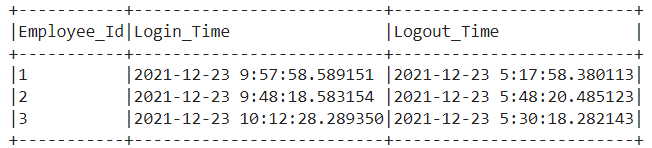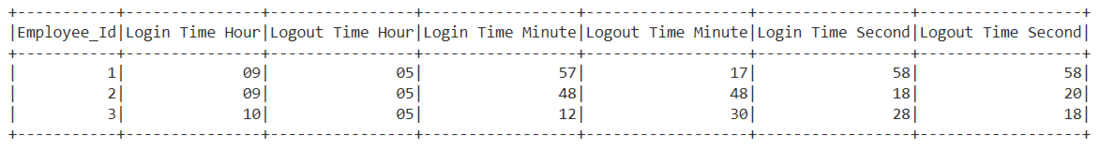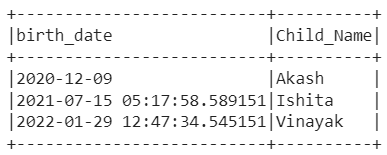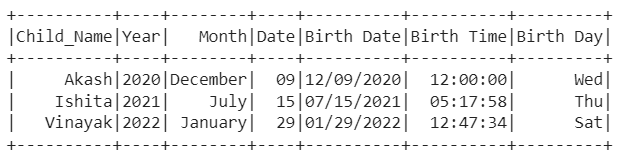GeeksforGeeks App
Open AppBrowser
Continue

# Partition of Timestamp column in Dataframes Pyspark

In this article, we are going to learn the partitioning of timestamp column in data frames using Pyspark in Python.

The timestamp column contains various time fields, such as year, month, week, day, hour, minute, second, millisecond, etc. There occurs various circumstances in which we don’t want all this information for our processing, what we need is just one or two fields. If we are stuck in a similar situation, then we can take use of date_format() function.

### date_format() function:

The function which will extract only the information we need in a column like an hour, minute, second, date, day, millisecond, day, month, or year and we can use it further in the data frame is known as date_format() function.

Syntax: date_format(date,format)

Parameters:

• date: It may be timestamp or timestamp column in the data frame that needs to be partitioned.
• format: It is the specific format in which you want to partition the column such as ‘YYYY’, ‘MM’, ‘DD’, ‘E’, etc.

### Stepwise Implementation of the partitioning of timestamp column in data frames using Pyspark:

Step 1: First of all, import the required libraries, i.e., SparkSession and functions. The SparkSession library is used to create the session while the functions library gives access to all built-in functions available in the Pyspark data frame.

```from pyspark.sql import SparkSession
from pyspark.sql.functions import *```

Step 2: Now, create a spark session using the getOrCreate() function

`spark_session = SparkSession.builder.getOrCreate()`

Step 3: Then, either create the data frame using the createDataFrame() function or read the CSV file using the read.csv() function

```data_frame=csv_file = spark_session.read.csv('#Path of CSV file',
sep = ',', inferSchema = True, header = True)```

or

```data_frame=spark_session.createDataFrame([(column_data_1), (column_data_2 ), (column_data_3 )],
['column_name_1', 'column_name_2','column_name_3'])```

Step 4: Finally, partition the timestamp columns using the date_format() function with the column name which needs to be partitioned and the format in which it needs to be partitioned as arguments. Also, you can use the alias function to give the fancy name to the partitioned column.

`df.select(date_format(df.column_name,"#format").alias("partitioned_column_name")).show()`

### Example 1:

In this example, we have created the data frame with three columns ‘Employee_Id’, ‘Login_Time’, and ‘Logout_Time’ as given below. Then, we are going to partition the ‘Login_Time’ and ‘Logout_Time’ columns using date_format() function to get login time hour, logout time hour, login time minute, logout time minute, login time second, and logout time second respectively.## Python3

 `# Import the SparkSession and functions library``from` `pyspark.sql ``import` `SparkSession``from` `pyspark.sql.functions ``import` `*`` ` `# Create a spark session using getOrCreate() function``spark_session ``=` `SparkSession.builder.getOrCreate()`` ` `# Create the data frame which needs to be partitioned``df ``=` `spark_session.createDataFrame(``    ``[(``1``, ``'2021-12-23 9:57:58.589151'``,``      ``'2021-12-23 5:17:58.380113'``),``     ``(``2``, ``'2021-12-23 9:48:18.583154'``,``      ``'2021-12-23 5:48:20.485123'``),``     ``(``3``, ``'2021-12-23 10:12:28.289350'``,``      ``'2021-12-23 5:30:18.282143'``)],``    ``[``'Employee_Id'``, ``'Login_Time'``, ``'Logout_Time'``])`` ` `# Partition of Login_Time and Logout_Time columns with hour, minute and second``df.select(df.Employee_Id,``          ``date_format(df.Login_Time,``                      ``"hh"``).alias(``"Login Time Hour"``),``          ``date_format(df.Logout_Time,``                      ``"hh"``).alias(``"Logout Time Hour"``),``          ``date_format(df.Login_Time,``                      ``"mm"``).alias(``"Login Time Minute"``),``          ``date_format(df.Logout_Time,``                      ``"mm"``).alias(``"Logout Time Minute"``),``          ``date_format(df.Login_Time,``                      ``"ss"``).alias(``"Login Time Second"``),``          ``date_format(df.Logout_Time,``                      ``"ss"``).alias(``"Logout Time Second"``)``          ``).show()`

Output:### Example 2:

In this example, we have created the data set with two columns ‘Child_Name’ and ‘Birth_Date’ as given below. Then, we are going to partition the ‘Birth_Date’ column using the date_format() function to get a year, month, date, birth date, birth time, and birthday respectively.## Python3

 `# Import the SparkSession and functions library``from` `pyspark.sql ``import` `SparkSession``from` `pyspark.sql.functions ``import` `*`` ` `# Create a spark session using getOrCreate() function``spark_session ``=` `SparkSession.builder.getOrCreate()`` ` `# Create the data frame which needs to be partitioned``df ``=` `spark_session.createDataFrame(``    ``[(``'2020-12-09'``, ``'Akash'``),``     ``(``'2021-07-15 05:17:58.589151'``, ``'Ishita'``),``        ``(``'2022-01-29 12:47:34.545151'``, ``'Vinayak'``)],``    ``[``'birth_date'``, ``'Child_Name'``])`` ` `# Partition of birth_date column with year, month,``# date, birth date, birth time and birth day``df.select(df.Child_Name,``          ``date_format(df.birth_date,``                      ``"yyyy"``).alias(``"Year"``),``          ``date_format(df.birth_date,``                      ``"MMMM"``).alias(``"Month"``),``          ``date_format(df.birth_date,``                      ``"dd"``).alias(``"Date"``),``          ``date_format(df.birth_date,``                      ``"MM/dd/yyyy"``).alias(``"Birth Date"``),``          ``date_format(df.birth_date,``                      ``"hh:mm:ss"``).alias(``"Birth Time"``),``          ``date_format(df.birth_date,``                      ``"E"``).alias(``"Birth Day"``)``          ``).show()`

Output:My Personal Notes arrow_drop_up